# niBT SG Channel Number to Carrier Frequency VI

NI Bluetooth Generation Toolkit 18.0 Help

Edition Date: January 2019

Part Number: 373432J-01

»View Product InfoDownload Help (Windows Only)

Computes the carrier frequency of the Bluetooth signal to generate based on the value that you specify in the channel number parameter. The toolkit computes the center frequency according to section 2, Part A, Volume 2 of the Bluetooth Specification Version 4.2.

The Bluetooth system operates in the 2.4 GHz ISM band. This frequency band is 2,400 MHz to 2,483.5 MHz. RF channels are spaced 1 MHz apart and are ordered in channel number k according to the following formula:

f = 2402+k MHz

where

k=0, 1, ..., 78 for Basic Rate/Enhanced Data Rate packets

f = 2402+(2*k) MHz

where

k=0, 1, ..., 39 for Low Energy packet

f is the frequency of the RF channels.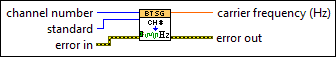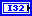channel number specifies the channel number.standard specifies the standard. The default value is Basic+EDR.

 Basic+EDR (0) Specifies that the standard is basic rate or enhanced data rate (EDR). LE (1) Specifies that the standard is low energy (LE).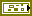error in describes error conditions that occur before this node runs. This input provides standard error in functionality.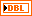carrier frequency returns the RF frequency (f) corresponding to the channel number k, where f = 2402 + k MHz.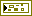error out contains error information. This output provides standard error out functionality.